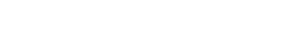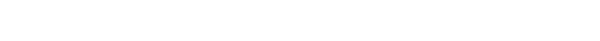## Resistance

 Listen to audio stream
Topic Progress:

The RESISTANCE of a conductor will depend on 4 things:

1. Length
Directly proportional to resistance. Double the length = double the resistance
2. CSA (conductor surface area)
Inversely proportional. Double the c.s.a = ½ the resistance
3. Temperature of the conductor
More heat, more resistance
4. Resistivity of the material
Every material has different properties, these include their ability to inhibit or facilitate the passage of current

### Using Ohm’s Law

#### Length = XResistance – 0.25Ω

#### Length = 2X (doubled)Resistance – 0.5Ω

#### CSA DoubledResistance – 0.125Ω

Think of current through a cable in the same way as you might think of water flowing through a pipe. It is clear that the narrower the pipe the harder it is for water to flow, the wider the pipe, the more water can flow.

### Resistance calculation example

Commonly used cables are 2.5mm2 and 1.5mm2 CSAIf a length of 2.5 mm2 csa cable has a resistance of 0.2Ω. What would the resistance of a 1.5 mm2 csa be of the same length?

(0.2 x 2.5)/1.5 = 0.2 x 1.67 = 0.334Ω

The csa has decreased by a factor of 1.67 so because csa and resistance are inversely proportional the resistance must increase by a factor of 1.67.

### Increase of resistance with temperature### Calculating Resistance in Series

Total resistance of a series of resistors = sum of each resistorRtotal = R1 + R2 + R3 + R4

If R1 = 5Ω, R2 = 5Ω, R3 = 5Ω, R4= 5Ω

Rtotal = 20Ω

### Calculating Resistance in Parallel

All circuits will have an insulation resistance and they are all connected in parallel at the supply cables.What is the value of these resistors in parallel?

There are three different methods of solving these problems. All result in the same answer

#### Method 1: The Fraction Method#### Method 3: The Product over Sum Method

This method can only be used on 2 values at a time, so:

First 2 resistors (10 x 20) / (10 + 20) = 6.66 r

Second 2 resistors (5 x 20) / (5 + 20) = 4

The two resultants (4 x 6.66r) / (4 + 6.66r) = 2.5 MΩ